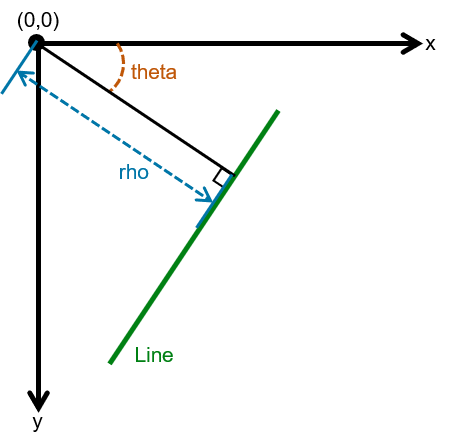# hough

Hough transform

## Syntax

[H,theta,rho] = hough(BW)
[H,theta,rho] = hough(BW,Name,Value)

## Description

example

[H,theta,rho] = hough(BW) computes the Standard Hough Transform (SHT) of the binary image BW. The hough function is designed to detect lines. The function uses the parametric representation of a line: rho = x*cos(theta) + y*sin(theta). The function returns rho, the distance from the origin to the line along a vector perpendicular to the line, and theta, the angle in degrees between the x-axis and this vector. The function also returns the SHT, H, which is a parameter space matrix whose rows and columns correspond to rho and theta values respectively. For more information, see Algorithms.

example

[H,theta,rho] = hough(BW,Name,Value) computes the SHT of the binary image BW using name-value arguments to affect the computation.

## Examples

collapse all

Read an image, and convert it to a grayscale image.

RGB = imread('gantrycrane.png'); I = im2gray(RGB);

Extract edges.

BW = edge(I,'canny');

Calculate Hough transform.

[H,T,R] = hough(BW,'RhoResolution',0.5,'Theta',-90:0.5:89);

Display the original image and the Hough matrix.

subplot(2,1,1); imshow(RGB); title('gantrycrane.png'); subplot(2,1,2); imshow(imadjust(rescale(H)),'XData',T,'YData',R,... 'InitialMagnification','fit'); title('Hough transform of gantrycrane.png'); xlabel('\theta'), ylabel('\rho'); axis on, axis normal, hold on; colormap(gca,hot);Read an image, and convert it to grayscale.

RGB = imread('gantrycrane.png'); I = im2gray(RGB);

Extract edges.

BW = edge(I,'canny');

Calculate the Hough transform over a limited range of angles.

[H,T,R] = hough(BW,'Theta',44:0.5:46);

Display the Hough transform.

figure imshow(imadjust(rescale(H)),'XData',T,'YData',R,... 'InitialMagnification','fit'); title('Limited Theta Range Hough Transform of Gantrycrane Image'); xlabel('\theta') ylabel('\rho'); axis on, axis normal; colormap(gca,hot)## Input Arguments

collapse all

Binary image, specified as a 2-D logical matrix or 2-D numeric matrix. For numeric input, any nonzero pixels are considered to be 1 (true).

Data Types: single | double | int8 | int16 | int32 | int64 | uint8 | uint16 | uint32 | uint64 | logical

### Name-Value Arguments

Specify optional pairs of arguments as Name1=Value1,...,NameN=ValueN, where Name is the argument name and Value is the corresponding value. Name-value arguments must appear after other arguments, but the order of the pairs does not matter.

Example: [H,theta,rho] = hough(BW,RhoResolution=0.5)

Before R2021a, use commas to separate each name and value, and enclose Name in quotes.

Example: [H,theta,rho] = hough(BW,"RhoResolution",0.5)

Spacing of Hough transform bins along the rho axis, specified as a positive number between 0 and norm(size(BW)), exclusive.

Data Types: double

Theta values for the SHT, specified as a numeric vector with elements in the range [-90, 90).

Example: -90:0.5:89.5

Data Types: double

## Output Arguments

collapse all

Hough transform matrix, returned as a numeric matrix of size nrho-by-ntheta. The rows and columns correspond to rho and theta values. For more information, see Algorithms.

Angle between the x-axis and the rho vector, in degrees, returned as a numeric matrix. For more information, see Algorithms.

Data Types: double

Distance from the origin to the line along a vector perpendicular to the line, returned as a numeric array. For more information, see Algorithms.

Data Types: double

## Algorithms

The Standard Hough Transform (SHT) uses the parametric representation of a line:

rho = x*cos(theta) + y*sin(theta)

The origin of the coordinate system is assumed to be at the center of the upper-left corner pixel.

The variable rho is the perpendicular distance from the origin to the line.

The variable theta is the angle of the perpendicular projection from the origin to the line, measured in degrees clockwise from the positive x-axis. The range of theta is –90° ≤ θ < 90°. The angle of the line itself is θ + 90°, also measured clockwise with respect to the positive x-axis.The SHT is a parameter space matrix whose rows and columns correspond to rho and theta values, respectively. The elements in the SHT represent accumulator cells. Initially, the value in each cell is zero. Then, for every non-background point in the image, rho is calculated for every theta. rho is rounded off to the nearest allowed row in SHT. That accumulator cell is incremented. At the end of this procedure, a value of Q in SHT(r,c) means that Q points in the xy-plane lie on the line specified by theta(c) and rho(r). Peak values in the SHT represent potential lines in the input image.

The Hough transform matrix, H, is nrho-by-ntheta where:

nrho = 2*(ceil(D/RhoResolution)) + 1, and
D = sqrt((numRowsInBW - 1)^2 + (numColsInBW - 1)^2).
rho values range from -diagonal to diagonal, where
diagonal = RhoResolution*ceil(D/RhoResolution).

ntheta = length(theta)

## Version History

Introduced before R2006a

expand all